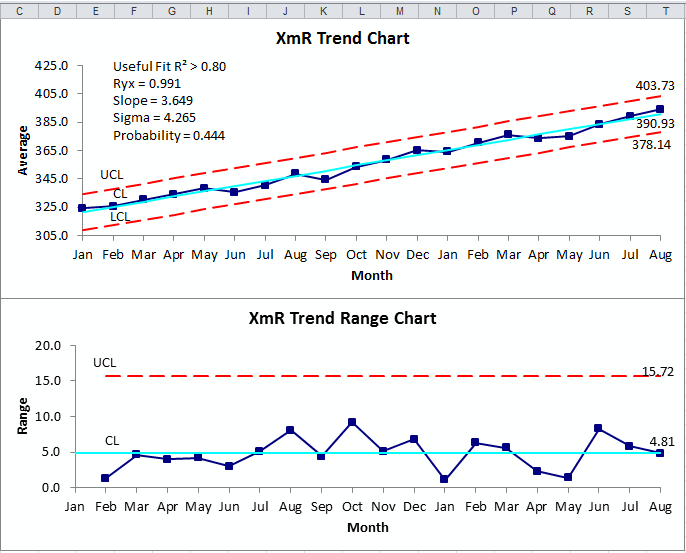# XmR Trend Control Chart in Excel

## Use an XmR Trend Control Chart to evaluate stability of processes using trended data - costs (e.g., inflation).

### XmR Trend Control Chart created using QI Macros for Excel.### The Chart will calculate the fit of the data to the trend:

Useful Fit R² > 0.80
Ryx = 0.991
Slope = 3.649
Sigma = 4.265
Probability = 0.444

• R² gives the fit of the line to the trend. Greater than 0.80 is a good fit.
• Ryx is the linear correlation coefficient. Compare to probability for df = n - 2.
• Slope is the positive or negative slope of the trend
• Sigma is the estimated standard deviation Rbar/d2
• Probability is critical value for Ryx. If Ryx > Probability then a statistically significant correlation exists.

### Why Choose QI Macros Over Other Control Chart Software?### Affordable

• Only \$329 USD - less with quantity discounts
• No annual subscription fees
• Free technical support### Easy to Use

• Works right in Excel
• Create a chart in seconds
• Easy to customize & share charts### Proven and Trusted

• More than 100,000 users
• In More than 80 countries
• Five Star CNET Rating - Virus free